# plot3

3-D point or line plot

•## Syntax

``plot3(X,Y,Z)``
``plot3(X,Y,Z,LineSpec)``
``plot3(X1,Y1,Z1,...,Xn,Yn,Zn)``
``plot3(X1,Y1,Z1,LineSpec1,...,Xn,Yn,Zn,LineSpecn)``
``plot3(___,Name,Value)``
``plot3(ax,___)``
``p = plot3(___)``

## Description

example

````plot3(X,Y,Z)` plots coordinates in 3-D space. To plot a set of coordinates connected by line segments, specify `X`, `Y`, and `Z` as vectors of the same length.To plot multiple sets of coordinates on the same set of axes, specify at least one of `X`, `Y`, or `Z` as a matrix and the others as vectors. ```

example

````plot3(X,Y,Z,LineSpec)` creates the plot using the specified line style, marker, and color.```

example

````plot3(X1,Y1,Z1,...,Xn,Yn,Zn)` plots multiple sets of coordinates on the same set of axes. Use this syntax as an alternative to specifying multiple sets as matrices.```

example

````plot3(X1,Y1,Z1,LineSpec1,...,Xn,Yn,Zn,LineSpecn)` assigns specific line styles, markers, and colors to each `XYZ` triplet. You can specify `LineSpec` for some triplets and omit it for others. For example, `plot3(X1,Y1,Z1,'o',X2,Y2,Z2)` specifies markers for the first triplet but not the for the second triplet.```

example

````plot3(___,Name,Value)` specifies `Line` properties using one or more name-value pair arguments. Specify the properties after all other input arguments. For a list of properties, see Line Properties.```

example

````plot3(ax,___)` displays the plot in the target axes. Specify the axes as the first argument in any of the previous syntaxes.```

example

````p = plot3(___)` returns a `Line` object or an array of `Line` objects. Use `p` to modify properties of the plot after creating it. For a list of properties, see Line Properties.```

## Examples

collapse all

Define `t` as a vector of values between 0 and 10$\pi$. Define `st` and `ct` as vectors of sine and cosine values. Then plot `st`, `ct`, and `t`.

```t = 0:pi/50:10*pi; st = sin(t); ct = cos(t); plot3(st,ct,t)```Create two sets of x-, y-, and z-coordinates.

```t = 0:pi/500:pi; xt1 = sin(t).*cos(10*t); yt1 = sin(t).*sin(10*t); zt1 = cos(t); xt2 = sin(t).*cos(12*t); yt2 = sin(t).*sin(12*t); zt2 = cos(t);```

Call the `plot3` function, and specify consecutive `XYZ` triplets.

`plot3(xt1,yt1,zt1,xt2,yt2,zt2)`Create matrix `X` containing three rows of x-coordinates. Create matrix `Y` containing three rows of y-coordinates.

```t = 0:pi/500:pi; X(1,:) = sin(t).*cos(10*t); X(2,:) = sin(t).*cos(12*t); X(3,:) = sin(t).*cos(20*t); Y(1,:) = sin(t).*sin(10*t); Y(2,:) = sin(t).*sin(12*t); Y(3,:) = sin(t).*sin(20*t);```

Create matrix `Z` containing the z-coordinates for all three sets.

`Z = cos(t);`

Plot all three sets of coordinates on the same set of axes.

`plot3(X,Y,Z)`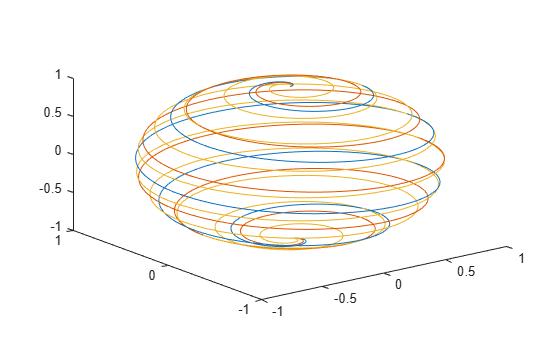Create vectors `xt`, `yt`, and `zt`.

```t = 0:pi/500:40*pi; xt = (3 + cos(sqrt(32)*t)).*cos(t); yt = sin(sqrt(32) * t); zt = (3 + cos(sqrt(32)*t)).*sin(t);```

Plot the data, and use the `axis equal` command to space the tick units equally along each axis. Then specify the labels for each axis.

```plot3(xt,yt,zt) axis equal xlabel('x(t)') ylabel('y(t)') zlabel('z(t)')```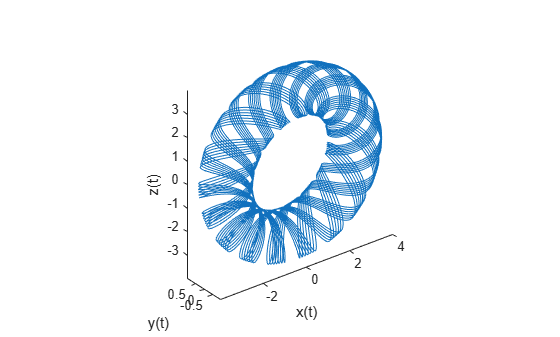Create vectors `t`, `xt`, and `yt`, and plot the points in those vectors using circular markers.

```t = 0:pi/20:10*pi; xt = sin(t); yt = cos(t); plot3(xt,yt,t,'o')```Create vectors `t`, `xt`, and `yt`, and plot the points in those vectors as a blue line with 10-point circular markers. Use a hexadecimal color code to specify a light blue fill color for the markers.

```t = 0:pi/20:10*pi; xt = sin(t); yt = cos(t); plot3(xt,yt,t,'-o','Color','b','MarkerSize',10,... 'MarkerFaceColor','#D9FFFF')```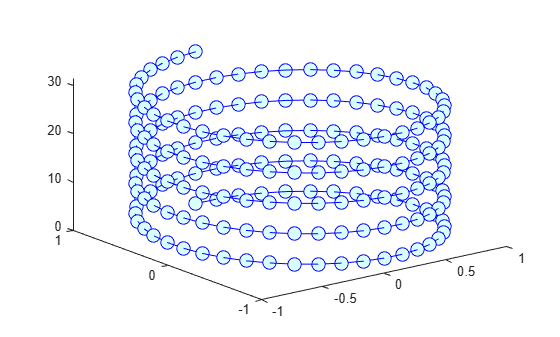Create vector `t`. Then use `t` to calculate two sets of x and y values.

```t = 0:pi/20:10*pi; xt1 = sin(t); yt1 = cos(t); xt2 = sin(2*t); yt2 = cos(2*t);```

Plot the two sets of values. Use the default line for the first set, and specify a dashed line for the second set.

`plot3(xt1,yt1,t,xt2,yt2,t,'--')`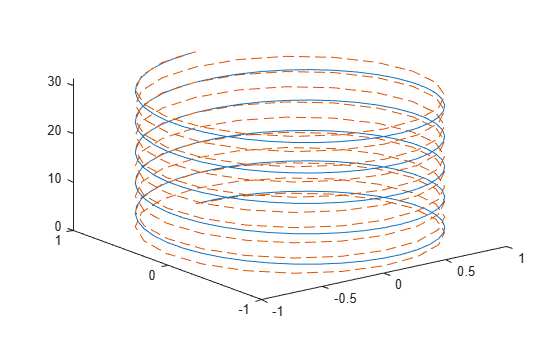Create vectors `t`, `xt`, and `yt`, and plot the data in those vectors. Return the chart line in the output variable `p`.

```t = linspace(-10,10,1000); xt = exp(-t./10).*sin(5*t); yt = exp(-t./10).*cos(5*t); p = plot3(xt,yt,t);```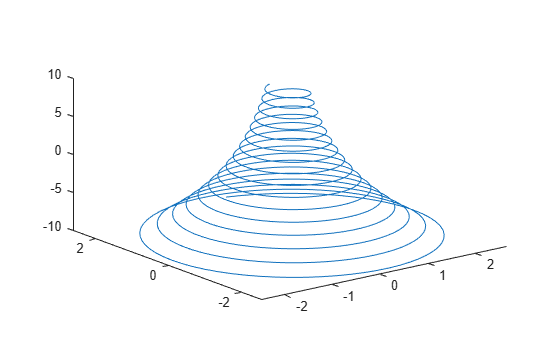Change the line width to `3`.

`p.LineWidth = 3;`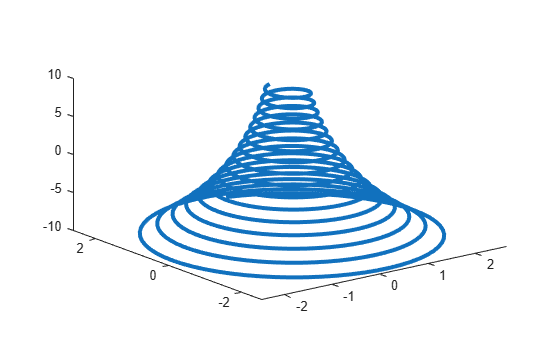Starting in R2019b, you can display a tiling of plots using the `tiledlayout` and `nexttile` functions. Call the `tiledlayout` function to create a 1-by-2 tiled chart layout. Call the `nexttile` function to create the axes objects `ax1` and `ax2`. Create separate line plots in the axes by specifying the axes object as the first argument to `plot`3.

```tiledlayout(1,2) % Left plot ax1 = nexttile; t = 0:pi/20:10*pi; xt1 = sin(t); yt1 = cos(t); plot3(ax1,xt1,yt1,t) title(ax1,'Helix With 5 Turns') % Right plot ax2 = nexttile; t = 0:pi/20:10*pi; xt2 = sin(2*t); yt2 = cos(2*t); plot3(ax2,xt2,yt2,t) title(ax2,'Helix With 10 Turns')```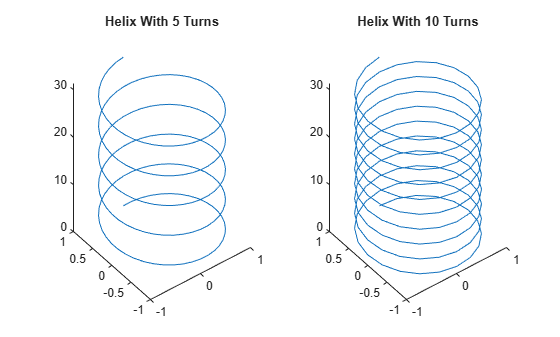Create `x` and `y` as vectors of random values between `0` and `1`. Create `z` as a vector of random duration values.

```x = rand(1,10); y = rand(1,10); z = duration(rand(10,1),randi(60,10,1),randi(60,10,1));```

Plot `x`, `y`, and `z`, and specify the format for the z-axis as minutes and seconds. Then add axis labels, and turn on the grid to make it easier to visualize the points within the plot box.

```plot3(x,y,z,'o','DurationTickFormat','mm:ss') xlabel('X') ylabel('Y') zlabel('Duration') grid on```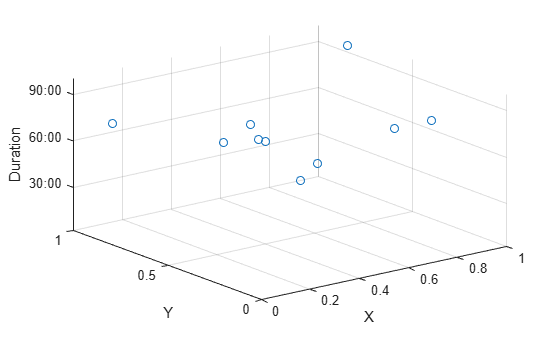Create vectors `xt`, `yt`, and `zt`. Plot the values, specifying a solid line with circular markers using the `LineSpec` argument. Specify the `MarkerIndices` property to place one marker at the 200th data point.

```t = 0:pi/500:pi; xt(1,:) = sin(t).*cos(10*t); yt(1,:) = sin(t).*sin(10*t); zt = cos(t); plot3(xt,yt,zt,'-o','MarkerIndices',200)```## Input Arguments

collapse all

x-coordinates, specified as a scalar, vector, or matrix. The size and shape of `X` depends on the shape of your data and the type of plot you want to create. This table describes the most common situations.

Type of PlotHow to Specify Coordinates
Single point

Specify `X`, `Y`, and `Z` as scalars and include a marker. For example:

`plot3(1,2,3,'o')`

One set of points

Specify `X`, `Y`, and `Z` as any combination of row or column vectors of the same length. For example:

`plot3([1 2 3],[4; 5; 6],[7 8 9])`

Multiple sets of points
(using vectors)

Specify consecutive sets of `X`, `Y`, and `Z` vectors. For example:

`plot3([1 2 3],[4 5 6],[7 8 9],[1 2 3],[4 5 6],[10 11 12])`

Multiple sets of points
(using matrices)

Specify at least one of `X`, `Y`, or `Z` as a matrix, and the others as vectors. Each of `X`, `Y`, and `Z` must have at least one dimension that is same size. For best results, specify all vectors of the same shape and all matrices of the same shape. For example:

`plot3([1 2 3],[4 5 6],[7 8 9; 10 11 12])`

Data Types: `single` | `double` | `int8` | `int16` | `int32` | `int64` | `uint8` | `uint16` | `uint32` | `uint64` | `categorical` | `datetime` | `duration`

y-coordinates, specified as a scalar, vector, or matrix. The size and shape of `Y` depends on the shape of your data and the type of plot you want to create. This table describes the most common situations.

Type of PlotHow to Specify Coordinates
Single point

Specify `X`, `Y`, and `Z` as scalars and include a marker. For example:

`plot3(1,2,3,'o')`

One set of points

Specify `X`, `Y`, and `Z` as any combination of row or column vectors of the same length. For example:

`plot3([1 2 3],[4; 5; 6],[7 8 9])`

Multiple sets of points
(using vectors)

Specify consecutive sets of `X`, `Y`, and `Z` vectors. For example:

`plot3([1 2 3],[4 5 6],[7 8 9],[1 2 3],[4 5 6],[10 11 12])`

Multiple sets of points
(using matrices)

Specify at least one of `X`, `Y`, or `Z` as a matrix, and the others as vectors. Each of `X`, `Y`, and `Z` must have at least one dimension that is same size. For best results, specify all vectors of the same shape and all matrices of the same shape. For example:

`plot3([1 2 3],[4 5 6],[7 8 9; 10 11 12])`

Data Types: `single` | `double` | `int8` | `int16` | `int32` | `int64` | `uint8` | `uint16` | `uint32` | `uint64` | `categorical` | `datetime` | `duration`

z-coordinates, specified as a scalar, vector, or matrix. The size and shape of `Z` depends on the shape of your data and the type of plot you want to create. This table describes the most common situations.

Type of PlotHow to Specify Coordinates
Single point

Specify `X`, `Y`, and `Z` as scalars and include a marker. For example:

`plot3(1,2,3,'o')`

One set of points

Specify `X`, `Y`, and `Z` as any combination of row or column vectors of the same length. For example:

`plot3([1 2 3],[4; 5; 6],[7 8 9])`

Multiple sets of points
(using vectors)

Specify consecutive sets of `X`, `Y`, and `Z` vectors. For example:

`plot3([1 2 3],[4 5 6],[7 8 9],[1 2 3],[4 5 6],[10 11 12])`

Multiple sets of points
(using matrices)

Specify at least one of `X`, `Y`, or `Z` as a matrix, and the others as vectors. Each of `X`, `Y`, and `Z` must have at least one dimension that is same size. For best results, specify all vectors of the same shape and all matrices of the same shape. For example:

`plot3([1 2 3],[4 5 6],[7 8 9; 10 11 12])`

Data Types: `single` | `double` | `int8` | `int16` | `int32` | `int64` | `uint8` | `uint16` | `uint32` | `uint64` | `categorical` | `datetime` | `duration`

Line style, marker, and color, specified as a character vector or string containing symbols. The symbols can appear in any order. You do not need to specify all three characteristics (line style, marker, and color). For example, if you omit the line style and specify the marker, then the plot shows only the marker and no line.

Example: `'--or'` is a red dashed line with circle markers

Line StyleDescriptionResulting Line
`'-'`Solid line`'--'`Dashed line`':'`Dotted line`'-.'`Dash-dotted lineMarkerDescriptionResulting Marker
`'o'`Circle`'+'`Plus sign`'*'`Asterisk`'.'`Point`'x'`Cross`'_'`Horizontal line`'|'`Vertical line`'s'`Square`'d'`Diamond`'^'`Upward-pointing triangle`'v'`Downward-pointing triangle`'>'`Right-pointing triangle`'<'`Left-pointing triangle`'p'`Pentagram`'h'`HexagramColor NameShort NameRGB TripletAppearance
`'red'``'r'``[1 0 0]``'green'``'g'``[0 1 0]``'blue'``'b'``[0 0 1]``'cyan'` `'c'``[0 1 1]``'magenta'``'m'``[1 0 1]``'yellow'``'y'``[1 1 0]``'black'``'k'``[0 0 0]``'white'``'w'``[1 1 1]`Target axes, specified as an `Axes` object. If you do not specify the axes and if the current axes is Cartesian, then `plot3` uses the current axes.

### Name-Value Arguments

Specify optional comma-separated pairs of `Name,Value` arguments. `Name` is the argument name and `Value` is the corresponding value. `Name` must appear inside quotes. You can specify several name and value pair arguments in any order as `Name1,Value1,...,NameN,ValueN`.

Example: `plot3([1 2],[3 4],[5 6],'Color','red')` specifies a red line for the plot.

Note

The properties listed here are only a subset. For a complete list, see Line Properties.

Color, specified as an RGB triplet, a hexadecimal color code, a color name, or a short name. The color you specify sets the line color. It also sets the marker edge color when the `MarkerEdgeColor` property is set to `'auto'`.

For a custom color, specify an RGB triplet or a hexadecimal color code.

• An RGB triplet is a three-element row vector whose elements specify the intensities of the red, green, and blue components of the color. The intensities must be in the range `[0,1]`; for example, ```[0.4 0.6 0.7]```.

• A hexadecimal color code is a character vector or a string scalar that starts with a hash symbol (`#`) followed by three or six hexadecimal digits, which can range from `0` to `F`. The values are not case sensitive. Thus, the color codes `'#FF8800'`, `'#ff8800'`, `'#F80'`, and `'#f80'` are equivalent.

Alternatively, you can specify some common colors by name. This table lists the named color options, the equivalent RGB triplets, and hexadecimal color codes.

Color NameShort NameRGB TripletHexadecimal Color CodeAppearance
`'red'``'r'``[1 0 0]``'#FF0000'``'green'``'g'``[0 1 0]``'#00FF00'``'blue'``'b'``[0 0 1]``'#0000FF'``'cyan'` `'c'``[0 1 1]``'#00FFFF'``'magenta'``'m'``[1 0 1]``'#FF00FF'``'yellow'``'y'``[1 1 0]``'#FFFF00'``'black'``'k'``[0 0 0]``'#000000'``'white'``'w'``[1 1 1]``'#FFFFFF'``'none'`Not applicableNot applicableNot applicableNo color

Here are the RGB triplets and hexadecimal color codes for the default colors MATLAB® uses in many types of plots.

`[0 0.4470 0.7410]``'#0072BD'``[0.8500 0.3250 0.0980]``'#D95319'``[0.9290 0.6940 0.1250]``'#EDB120'``[0.4940 0.1840 0.5560]``'#7E2F8E'``[0.4660 0.6740 0.1880]``'#77AC30'``[0.3010 0.7450 0.9330]``'#4DBEEE'``[0.6350 0.0780 0.1840]``'#A2142F'`Line width, specified as a positive value in points, where 1 point = 1/72 of an inch. If the line has markers, then the line width also affects the marker edges.

The line width cannot be thinner than the width of a pixel. If you set the line width to a value that is less than the width of a pixel on your system, the line displays as one pixel wide.

Marker size, specified as a positive value in points, where 1 point = 1/72 of an inch.

Marker outline color, specified as `'auto'`, an RGB triplet, a hexadecimal color code, a color name, or a short name. The default value of `'auto'` uses the same color as the `Color` property.

For a custom color, specify an RGB triplet or a hexadecimal color code.

• An RGB triplet is a three-element row vector whose elements specify the intensities of the red, green, and blue components of the color. The intensities must be in the range `[0,1]`; for example, ```[0.4 0.6 0.7]```.

• A hexadecimal color code is a character vector or a string scalar that starts with a hash symbol (`#`) followed by three or six hexadecimal digits, which can range from `0` to `F`. The values are not case sensitive. Thus, the color codes `'#FF8800'`, `'#ff8800'`, `'#F80'`, and `'#f80'` are equivalent.

Alternatively, you can specify some common colors by name. This table lists the named color options, the equivalent RGB triplets, and hexadecimal color codes.

Color NameShort NameRGB TripletHexadecimal Color CodeAppearance
`'red'``'r'``[1 0 0]``'#FF0000'``'green'``'g'``[0 1 0]``'#00FF00'``'blue'``'b'``[0 0 1]``'#0000FF'``'cyan'` `'c'``[0 1 1]``'#00FFFF'``'magenta'``'m'``[1 0 1]``'#FF00FF'``'yellow'``'y'``[1 1 0]``'#FFFF00'``'black'``'k'``[0 0 0]``'#000000'``'white'``'w'``[1 1 1]``'#FFFFFF'``'none'`Not applicableNot applicableNot applicableNo color

Here are the RGB triplets and hexadecimal color codes for the default colors MATLAB uses in many types of plots.

`[0 0.4470 0.7410]``'#0072BD'``[0.8500 0.3250 0.0980]``'#D95319'``[0.9290 0.6940 0.1250]``'#EDB120'``[0.4940 0.1840 0.5560]``'#7E2F8E'``[0.4660 0.6740 0.1880]``'#77AC30'``[0.3010 0.7450 0.9330]``'#4DBEEE'``[0.6350 0.0780 0.1840]``'#A2142F'`Marker fill color, specified as `'auto'`, an RGB triplet, a hexadecimal color code, a color name, or a short name. The `'auto'` option uses the same color as the `Color` property of the parent axes. If you specify `'auto'` and the axes plot box is invisible, the marker fill color is the color of the figure.

For a custom color, specify an RGB triplet or a hexadecimal color code.

• An RGB triplet is a three-element row vector whose elements specify the intensities of the red, green, and blue components of the color. The intensities must be in the range `[0,1]`; for example, ```[0.4 0.6 0.7]```.

• A hexadecimal color code is a character vector or a string scalar that starts with a hash symbol (`#`) followed by three or six hexadecimal digits, which can range from `0` to `F`. The values are not case sensitive. Thus, the color codes `'#FF8800'`, `'#ff8800'`, `'#F80'`, and `'#f80'` are equivalent.

Alternatively, you can specify some common colors by name. This table lists the named color options, the equivalent RGB triplets, and hexadecimal color codes.

Color NameShort NameRGB TripletHexadecimal Color CodeAppearance
`'red'``'r'``[1 0 0]``'#FF0000'``'green'``'g'``[0 1 0]``'#00FF00'``'blue'``'b'``[0 0 1]``'#0000FF'``'cyan'` `'c'``[0 1 1]``'#00FFFF'``'magenta'``'m'``[1 0 1]``'#FF00FF'``'yellow'``'y'``[1 1 0]``'#FFFF00'``'black'``'k'``[0 0 0]``'#000000'``'white'``'w'``[1 1 1]``'#FFFFFF'``'none'`Not applicableNot applicableNot applicableNo color

Here are the RGB triplets and hexadecimal color codes for the default colors MATLAB uses in many types of plots.

`[0 0.4470 0.7410]``'#0072BD'``[0.8500 0.3250 0.0980]``'#D95319'``[0.9290 0.6940 0.1250]``'#EDB120'``[0.4940 0.1840 0.5560]``'#7E2F8E'``[0.4660 0.6740 0.1880]``'#77AC30'``[0.3010 0.7450 0.9330]``'#4DBEEE'``[0.6350 0.0780 0.1840]``'#A2142F'`## Tips

• Use `NaN` or `Inf` to create breaks in the lines. For example, this code plots a line with a break between `z=2` and `z=4`.

` plot3([1 2 3 4 5],[1 2 3 4 5],[1 2 NaN 4 5])`

• `plot3` uses colors and line styles based on the `ColorOrder` and `LineStyleOrder` properties of the axes. `plot3` cycles through the colors with the first line style. Then, it cycles through the colors again with each additional line style.

Starting in R2019b, you can change the colors and the line styles after plotting by setting the `ColorOrder` or `LineStyleOrder` properties on the axes. You can also call the `colororder` function to change the color order for all the axes in the figure.

## Extended Capabilities

### Topics

Introduced before R2006a